# Goyal Brothers Prakashan solutions for Class 9 A New Approach to ICSE Physics Part 1 chapter 8 - Light [Latest edition]

#### Chapters## Chapter 8: Light

Unit I Exercise IUnit II Practice Problem 1Unit II Practice Problem 2Unit II Practice Problem 3Exercise II Objective QuestionsExercise II Subjective Questions
Unit I Exercise I

### Goyal Brothers Prakashan solutions for Class 9 A New Approach to ICSE Physics Part 1 Chapter 8 Light Unit I Exercise I

Unit I Exercise I | Q 1.1

What do you understand by the Diffused Light?

Unit I Exercise I | Q 1.1

What do you understand by the Light?

Unit I Exercise I | Q 1.2

What is irregular reflection ? Give an example.

Unit I Exercise I | Q 1.2

By giving one example and one use explain or define Regular reflection.

Unit I Exercise I | Q 2.1

By drawing a neat diagram define the following:

Mirror

Unit I Exercise I | Q 2.2

Explain the following term:

Incident ray

Draw diagram/diagrams to show them.

Unit I Exercise I | Q 2.3

Explain the following term:

Reflected ray

Draw diagram/diagrams to show them.

Unit I Exercise I | Q 2.4

Explain the following term:

Angle of incidence

Draw diagram/diagrams to show them.

Unit I Exercise I | Q 2.5

Explain the following term:

Angle of reflection.

Draw diagram/diagrams to show them.

Unit I Exercise I | Q 2.6

Explain the following term:

Normal Draw

diagram/diagrams to show them.

Unit I Exercise I | Q 3

State the laws of reflection.

Unit I Exercise I | Q 4

A ray of light strikes a plane mirror, such that the angle with the mirror is 20°. What is the value of angle of reflection? What is the angle between the incident ray and the reflected ray?

Unit I Exercise I | Q 5

Prove experimentally that images are formed as far behind in a plane mirror as the object is in front of it.

Unit I Exercise I | Q 6

Prove geometrically that when plane mirror turns through a certain angle, the reflected ray turns through twice the angle.

Unit I Exercise I | Q 7

(a) What do you understand by the term lateral inversion?
(b) A printed card has letters PHYSICS. By drawing the diagram show the appearance of the letters. (No ray diagram is required).

Unit I Exercise I | Q 8.1

State the mirror formula for the formation of total number of images formed in two plane mirrors, held at an angle.

Unit I Exercise I | Q 8.2

Calculate the number of images formed in two plane mirrors, when they are held at the angle of (i) 72° (ii) 36°.

Unit I Exercise I | Q 9.1

Draw a neat two ray diagram for the formation of images in two plane mirrors, when mirrors are at right angles to each other.

Unit I Exercise I | Q 9.2

Draw a neat two ray diagram for the formation of images in two plane mirrors, when mirrors are at facing each other.

Unit I Exercise I | Q 10

Why are infinite images not seen when two plane mirrors are facing each other?

Unit I Exercise I | Q 11.1

State four characteristics of the image formed by a plane mirror.

Unit I Exercise I | Q 11.2

State three ways in which the image formed in a plane mirror differs from the image formed in a pin hole camera.

Unit I Exercise I | Q 12.1

What should be the minimum size of a plane mirror, so that a person 182 cm high can see himself completely?

Unit I Exercise I | Q 12.2

A boy stands 4 m away from the plane mirror. If the boy moves 1/2 m towards the mirror, what is now the distance between the boy and his image? Give a reason for your answer.

Unit I Exercise I | Q 13

State four uses of a plane mirror.

Unit I Exercise I | Q 14.1

Draw a neat diagram of reflecting periscope.

Unit I Exercise I | Q 14.2

State two advantages and two disadvantages of the reflecting periscope.

Unit I Exercise I | Q 15

What must be the minimum length of a plane mirror in which a person can see himself full length? Draw a diagram to justify your answer. Does the distance of a person from the mirror affect the above answer?

Unit I Exercise I | Q 16

An insect is sitting in front of a plane mirror at a distance of one meter from it.

1. Where is the image of insect formed?
2. What is the distance between insect and its image?
3. State any two characteristics of image formed in a plane mirror.
Unit I Exercise I | Q 17.1

Draw a diagram to show the reflection of a ray of light using a plane mirror. In the diagram, label the incident ray, the reflected ray, the normal, the angle of incidence and the angle of reflection.

Unit I Exercise I | Q 17.2

State the laws of reflection.

Unit I Exercise I | Q 18.1

Parallel rays are incident:

1. on regular surface and
2. on irregular surface. In what respect do reflected rays in (1) differ from those of (2)?
Unit I Exercise I | Q 18.2

Write down four characteristics of image formed in a plane mirror.

Unit I Exercise I | Q 19

How many images will be formed when an object is placed between two parallel plane mirrors with their reflecting surfaces facing each other? Why do more distant images appear fainter?

Unit I Exercise I | Q 20.1

Write down the letters of the word ‘POLEX’ as seen in a plane mirror, held parallel to the plane of this paper.

Unit I Exercise I | Q 20.2

Distinguish between real and virtual image.

Unit I Exercise I | Q 21.1

Describe the principle of simple periscope through an outline ray diagram. Give one of its uses.

Unit I Exercise I | Q 21.2

Draw diagrams to show the difference between regular and irregular reflection.

Unit I Exercise I | Q 22

An object is placed 2 cm from a plane mirror. If the object is moved by 1 cm towards the mirror, what will be the distance between the object and its new image?

Unit II Practice Problem 1

### Goyal Brothers Prakashan solutions for Class 9 A New Approach to ICSE Physics Part 1 Chapter 8 Light Unit II Practice Problem 1

Unit II Practice Problem 1 | Q 1

An object 3 cm high produces a real image 4.5 cm high when placed at a distance of 20 cm from a concave mirror. Calculate:

1. the position of image
2. focal length of the concave mirror.
Unit II Practice Problem 2

### Goyal Brothers Prakashan solutions for Class 9 A New Approach to ICSE Physics Part 1 Chapter 8 Light Unit II Practice Problem 2

Unit II Practice Problem 2 | Q 1

An object 1.5 cm high when placed in front of a concave. the mirror produces a virtual image 3 cm high. If the object is placed at a distance of 6 cm from the pole of the mirror, calculate:

1. the position of the image
2. the focal length of the mirror.
Unit II Practice Problem 2 | Q 2

A converging mirror, forms three times magnified virtual image when an object is placed at a distance of 8 cm from it. Calculate:

1. the position of the image
2. the focal length of the mirror.
Unit II Practice Problem 3

### Goyal Brothers Prakashan solutions for Class 9 A New Approach to ICSE Physics Part 1 Chapter 8 Light Unit II Practice Problem 3

Unit II Practice Problem 3 | Q 1

An object 5 cm high forms a virtual image of 1.25 cm high, when placed in front of a convex mirror at a distance of 24 cm. Calculate:

1. the position of the image
2. the focal length of the convex mirror.
Unit II Practice Problem 3 | Q 2

An object forms a virtual image which is 1/8th of the size of the object. If the object is placed at a distance of 40 cm from the convex mirror, calculate:

1. the position of the image
2. the focal length of the convex mirror.
Exercise II Objective Questions

### Goyal Brothers Prakashan solutions for Class 9 A New Approach to ICSE Physics Part 1 Chapter 8 Light Exercise II Objective Questions

Exercise II Objective Questions | Q 1

Select the correct option:

A concave mirror is made by cutting a portion of a hollow glass sphere of radius 30 cm. The focal length of the concave mirror is:

• 24 cm

• 12 cm

• 15 cm

• 60 cm

Exercise II Objective Questions | Q 2

Select the correct option:

A mirror forms a virtual image (diminished) of an object, whatever be the position of object:

• it must be a concave mirror

• it must be a convex mirror

• it must be a plane mirror

• it may be convex mirror or plane mirror

Exercise II Objective Questions | Q 3

Select the correct option:

A ray of light is incident on a concave mirror. If it is parallel to principal axis, the reflected ray will:

• pass through its principal focus

• pass through its centre of curvature

• pass through its pole

• retraces its path

Exercise II Objective Questions | Q 4

Select the correct option:

If an incident ray passes through the centre of curvature of a spherical mirror, the reflected ray will:

• pass through its pole

• retraces its path

• pass through its focus

• be parallel to principal axis

Exercise II Objective Questions | Q 5

Select the correct option:

In case of concave mirror, the minimum distance between an object and its real image is:

• f

• 2f

• 4f

• zero

Exercise II Objective Questions | Q 6

Select the correct option:

Looking into a mirror one finds her image diminished, the mirror is:

• concave

• convex

• cylindrical

• parabolic

Exercise II Objective Questions | Q 7

Select the correct option:

Which mirror is used in periscope?

• concave mirror

• convex mirror

• Plane mirror

• parabolic mirror

Exercise II Subjective Questions

### Goyal Brothers Prakashan solutions for Class 9 A New Approach to ICSE Physics Part 1 Chapter 8 Light Exercise II Subjective Questions

Exercise II Subjective Questions | Q 1.1

Define the following term:

spherical mirror

Exercise II Subjective Questions | Q 1.2

Define the following term:

convex mirror

Exercise II Subjective Questions | Q 1.3

Define the following term:

concave mirror

Exercise II Subjective Questions | Q 2.1

Define the following term in relation to concave mirror.

Pole

Exercise II Subjective Questions | Q 2.2

Define the following term in relation to concave mirror.

Center of curvature

Exercise II Subjective Questions | Q 2.3

Define the following term in relation to concave mirror.

Principal axis

Exercise II Subjective Questions | Q 2.4

Define the following term in relation to concave mirror.

Principal focus

Exercise II Subjective Questions | Q 2.5

Define the following term in relation to concave mirror.

Focal length

Exercise II Subjective Questions | Q 2.6

Define the following term in relation to concave mirror.

Exercise II Subjective Questions | Q 2.7

Define the following term in relation to concave mirror.

Aperture

Exercise II Subjective Questions | Q 3.1

Define the term principal focus in case of convex mirror. Draw a convex mirror and show its principal focus and focal length clearly.

Exercise II Subjective Questions | Q 3.2

What is the relation between focal length and radius of curvature of a concave mirror?

Exercise II Subjective Questions | Q 4.1

What do you understand by the term real image?

Exercise II Subjective Questions | Q 4.2

What type of mirror can be used to obtain a real image of an object?

Exercise II Subjective Questions | Q 4.3

Does the mirror name by your form a real image for all locations? Give a reason for your answer.

Exercise II Subjective Questions | Q 4.4

Is real image always inverted?

Exercise II Subjective Questions | Q 5

Copy the figure. By taking two rays from point A, show the formation of image. State four characteristics of the image.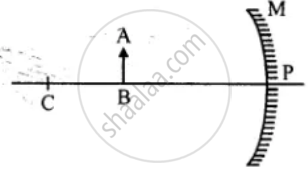Exercise II Subjective Questions | Q 6

Draw a neat two ray diagram to illustrate how a concave mirror is used as a shaving mirror.

Exercise II Subjective Questions | Q 7

Copy the figure. By taking two rays from point A, show the formation of image. State four characteristics of the image.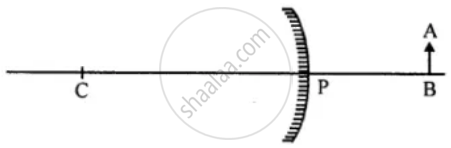Exercise II Subjective Questions | Q 8

Why do automobile drivers prefer convex mirror as a rear vew mirror? Illustrate your answer.

Exercise II Subjective Questions | Q 9.1

Give two uses of convex mirror.

Exercise II Subjective Questions | Q 9.2

Give two uses of concave mirror.

Exercise II Subjective Questions | Q 10

You are provided a convex mirror, a concave mirror and a plane mirror. How will you distinguish between them, without touching or using any other apparatus?

Exercise II Subjective Questions | Q 11

Compare the characteristics of an image formed by a convex mirror and a concave mirror, when object is beyond center of curvature, but not at infinity.

Exercise II Subjective Questions | Q 12

Why does a driver use a convex mirror instead of a plane mirror as a rear view mirror?
Illustrate your answer with the help of a ray diagram.

Exercise II Subjective Questions | Q 13.1

What is a real image?

Exercise II Subjective Questions | Q 13.2

What type of mirror is used to obtain a real image-of an object?

Exercise II Subjective Questions | Q 13.3

Does the mirror named by you above give real images for all locations of object?

Exercise II Subjective Questions | Q 14

In the figure is shown a concave mirror. A is a point on the principal axis. If an object O is kept at A, image is formed on A itself. Copy the diagram. Draw the image in the diagram. Is the image real or virtual?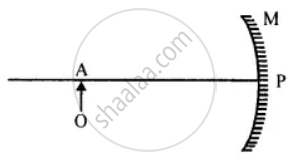Measure the distance PA and write it in the diagram. What is the distance PA called?
Mark a point B on the principal axis, at which, if a point source of light is kept, the rays travel parallel to principal axis after reflection from M. What is point B called?

Exercise II Subjective Questions | Q 15

An object OA is placed on the principal axis of a concave mirror as shown in the figure. Copy and complete the diagram to show the formation of image.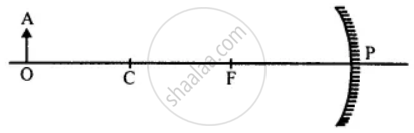Exercise II Subjective Questions | Q 16

Copy the figure and complete it, by drawing two rays to show the formation of the image of the object AB. State the size, position and nature of image formed.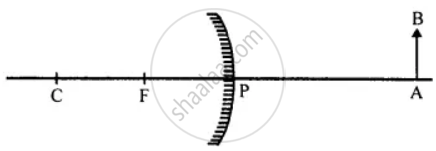## Chapter 8: Light

Unit I Exercise IUnit II Practice Problem 1Unit II Practice Problem 2Unit II Practice Problem 3Exercise II Objective QuestionsExercise II Subjective Questions## Goyal Brothers Prakashan solutions for Class 9 A New Approach to ICSE Physics Part 1 chapter 8 - Light

Goyal Brothers Prakashan solutions for Class 9 A New Approach to ICSE Physics Part 1 chapter 8 (Light) include all questions with solution and detail explanation. This will clear students doubts about any question and improve application skills while preparing for board exams. The detailed, step-by-step solutions will help you understand the concepts better and clear your confusions, if any. Shaalaa.com has the CISCE Class 9 A New Approach to ICSE Physics Part 1 solutions in a manner that help students grasp basic concepts better and faster.

Further, we at Shaalaa.com provide such solutions so that students can prepare for written exams. Goyal Brothers Prakashan textbook solutions can be a core help for self-study and acts as a perfect self-help guidance for students.

Concepts covered in Class 9 A New Approach to ICSE Physics Part 1 chapter 8 Light are Rules for the Construction of Image Formed by a Spherical Mirror, Focus and Focal Length, Images Formed by Spherical Mirrors, Concave Mirror, Image Formation by Concave Mirror, Convex Mirror, Mirror Equation/Formula, Images Formed by a Plane Mirrors, Distinction Between a Plane Mirror, Concave Mirror and Convex Mirror, Images Formed in a Pair of Mirrors Placed Parallel to Each Other, Images Formed by Two Mirrors Placed Perpendicular to Each Other, Spherical Mirrors, Image Formation by Convex Mirror, Relationship Between the Focal Length and Radius of Curvature, Sign Convention for Reflection by Spherical Mirrors, Reflection of Light, Types of Reflection, Terms Used in Reflection of Light, Law of Reflection of Light, Verification of the Law of Reflection of Light, Formation of Image by Reflection: Real and Virtual Image, Formation of Image of a Point Object by a Plane Mirror, Image of an Extended Object Formed by a Plane Mirror, Position of Image, Lateral Inversion, Plane Mirror and Reflection, Images Formed in Two Inclined Mirrors.

Using Goyal Brothers Prakashan Class 9 solutions Light exercise by students are an easy way to prepare for the exams, as they involve solutions arranged chapter-wise also page wise. The questions involved in Goyal Brothers Prakashan Solutions are important questions that can be asked in the final exam. Maximum students of CISCE Class 9 prefer Goyal Brothers Prakashan Textbook Solutions to score more in exam.

Get the free view of chapter 8 Light Class 9 extra questions for Class 9 A New Approach to ICSE Physics Part 1 and can use Shaalaa.com to keep it handy for your exam preparation